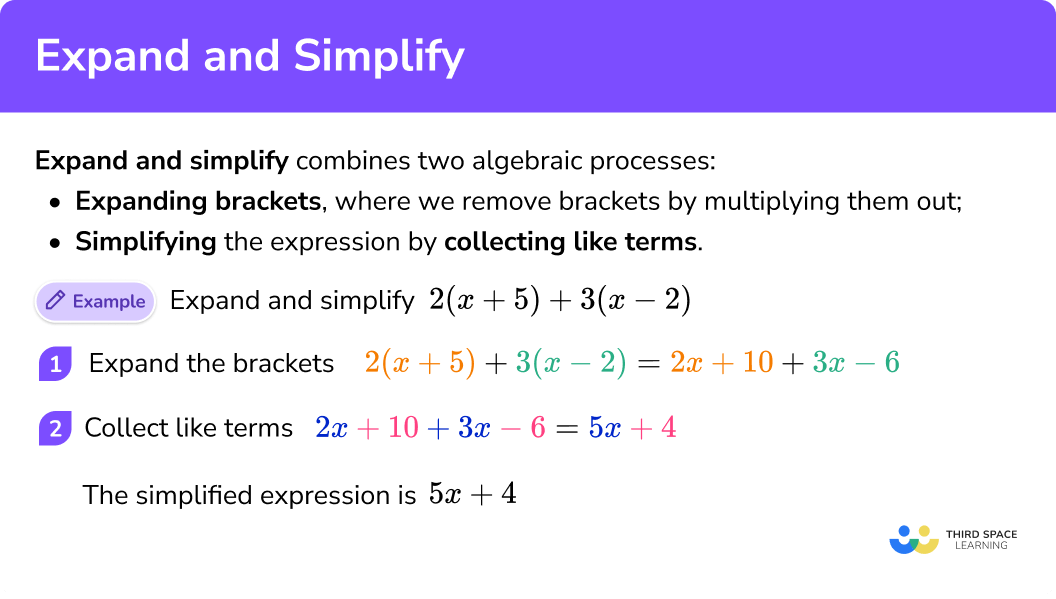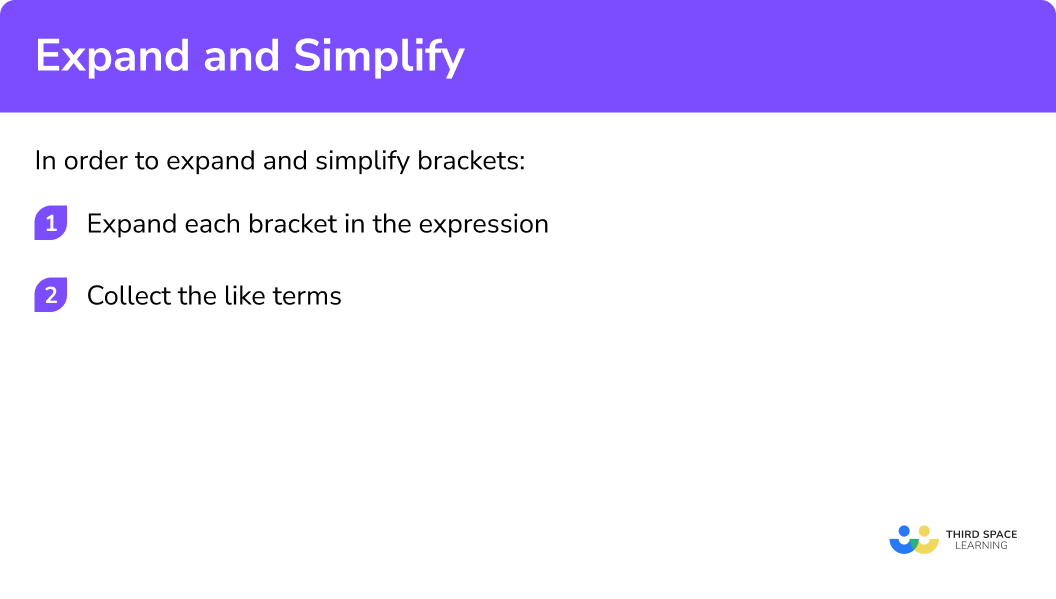# Expand And Simplify

Here we will learn how to expand and simplify algebraic expressions. First we expand the brackets, then we collect the like terms to simplify the expression.

At the end you’ll find expanding brackets worksheets based on Edexcel, AQA and OCR exam questions, along with further guidance on where to go next if you’re still stuck.

## What does ‘expand and simplify’ mean?

In order to expand and simplify an expression, we need to multiply out the brackets and then simplify the resulting expression by collecting the like terms.

Expanding brackets (or multiplying out) is the process by which we remove brackets.

It is the reverse process of factorisation. To expand brackets we multiply everything outside of the bracket, by everything inside of the bracket.

Once we have expanded the brackets we can simplify the expression by collecting the like terms.

E.g.

If we expand and simplify

2(x+5)+3(x-2)

we will get

\begin{aligned} 2(x+5)+3(x-2) &=2 x+10+3 x-6 \\\\ &=5 x+4 \end{aligned}

### What does expand and simplify mean?This lesson is part of our series of lessons to support revision on algebraic expressions. Related step by step guides include:

## Multiplying out brackets and simplify

‘Multiplying out brackets’ is another term for expanding brackets. It means exactly the same thing. “Expand the brackets” is the same as “multiply out the brackets”, it just gives the additional clue that when we expand brackets, we are multiplying everything outside the brackets by everything inside the brackets.

## How to expand and simplify brackets

In order to expand and simplify brackets:

1. Expand each bracket in the expression.
2. Collect the like terms.

There are three ways to expand and simplify brackets as covered below:

• single brackets
• two or more brackets
• surds

### Explain how to expand and simplify brackets?1. Expand and simplify with single brackets.

Expand the brackets to give the following expression:

E.g. 2(x + 5) + 3(x − 1)
= 2x + 10 + 3x − 3)
= 5x + 7

Remember: expressions with two terms like 5x + 7 are known as binomials.

2Expand and simplify with two or more brackets.

Expand the brackets to give the following expression:

E.g. (x + 5)(x − 1)
= x2 + 5x − x − 5
= x2 + 4x − 5

Remember: expressions with three terms like x2 + 4x − 5 are known as trinomials.

An expression that contains more than two terms and includes variables and coefficients is called a polynomial.

3 Expand and simplify with surds.

E.g. (3 + √5)(2 + √5)
= 6 + 3√5 + 2√5 + √5√5
= 11 + 5√5

## 1) Expand and simplify with single brackets

To expand a single bracket we multiply the term outside of the bracket by everything inside of the bracket. We can simplify the expression by collecting the like terms.

## Expand and simplify examples (with single brackets)

### Example 1: constants outside of the brackets

Expand and simplify:

2(x + 5) + 3(x − 2)

1. Expand each bracket in the expression

Multiply the first bracket:

Multiply the second bracket – remember we are multiplying both x and − 2 by + 3:

2(x + 5) + 3(x − 2)

= 2x + 10 + 3x − 6

2Collect the like terms

Highlight the two x terms (2x and + 3x) and the two constants (+ 10 and − 6).

2x + 10 + 3x − 6

2x + 3x = 5x
10 − 6 = + 4

= 5x + 4

### Example 2: constants and variables outside of the brackets

Expand and simplify:

2x(x + 6) - 3(x - 2)

Expand each bracket in the expression.

Collect the like terms.

### Example 3: variables in both terms in the brackets

Expand and simplify:

3(2x − 6y) − 5(x − 2y)

Expand each bracket in the expression.

Collect the like terms.

## Practice expand and simplify questions (with single brackets)

1. Expand and Simplify: 3(x+7)-2(x+3)

5x+15x+15x+275x+273(x+7)-2(x+3)

Expand each bracket

3x+21-2x-6

Collect like terms

x+15

2. Expand and simplify: 8(y-5)-5(y-2)

13y-303y-503y-3013y-508(y-5)-5(y-2)

Expand each bracket

8y-40-5y+10

Collect like terms

3y-30

3. Expand and simplify: 5x(3x-2)-4x(2x+3)

23x^{2}-22x7x^{2}-57x^{2}-22x7x^{2}-2x5x(3x-2)-4x(2x+3)

Expand each bracket

15x^{2}-10x-8x^{2}-12x

Collect like terms

7x^{2}-22x

4. Expand and simplify: 5(6x-2y)-2(8x-5y)

14x14x-20y46x14x+20y5(6x-2y)-2(8x-5y)

Expand each bracket

30x-10y-16x+10y

Collect like terms

14x

## 2) Expand and simplify with two or more brackets

To expand two or more brackets we multiply every term in the first bracket by every term in each of the other brackets.

## Expand and simplify examples (with two or more brackets)

### Example 1: variables have a coefficient of 1

Expand and simplify:

(x + 5)(x − 1)

1. Expand the brackets in the expression.

x ✕ x = x2
x ✕ − 1 = − x

+ = so the answer is negative.

x ✕ 5 = 5x
5 ✕ − 1 = − 5

+ = so the answer is negative.

(x + 5)(x − 1) = x2 − x + 5x − 5

2Collect the like terms.

The only like terms we have are the two x terms (− x and + 5x). Highlight them both.

x2 − x + 5x − 5

= x2 + 4x − 5

### Example 2: variables have a coefficient of greater than 1

Expand and simplify:

(2x − 4)(x + 5)

Expand the brackets in the expression.

Collect the like terms.

### Example 3: with triple brackets

Expand and simplify:

(x + 3)2(x − 1)

Expand and simplify the first two brackets in the expression.

Multiply this new expression with the third bracket and then simplify by collecting like terms.

## Practice expand and simplify questions (with two or more brackets)

1. Expand and simplify: (x+3)(x-7)

x^{2}-4x+21x^{2}-10x-21x^{2}-4x-21x^{2}-10x+21(x+3)(x-7)

Expand the brackets

x^{2}-7x+3x-21

and collect like terms

x^{2}-4x-21

2. Expand and simplify: (2x-4)(x-9)

2x^{2}-14x+362x^{2}-14x-362x^{2}-22x+362x^{2}-22x-36(2x-4)(x-9)

Expand the brackets

2x^{2}-18x-4x+36

and collect like terms

2x^{2}-22x+36

3. Expand and simplify: (x-3)^2(2x+1)

2x^{3}-13x^{2}+12x+92x^{3}+13x^{2}+12x+92x^{3}-11x^{2}+12x+92x^{3}-11x^{2}-24x+9(x-3)^{2}(2x+1)

can be written as
(x-3)(x-3)(2x+1)

Expanding the first two brackets gives

(x^{2}-3x-3x+9)(2x+1)

(x^{2}-6x+9)(2x+1)

then expanding again

2x^{3}+x^{2}-12x^{2}-6x+18x+9

and collecting like terms

2x^{3}-11x^{2}+12x+9

4. Expand and simplify: (2x-1)^{3}

8x^{3}+8x^{2}+6x+18x^{3}+12x^{2}+12x+18x^{3}-12x^{2}+6x-12x^{3}+12x^{2}+6x+1(2x-1)^{3}

can be written as
(2x-1)(2x-1)(2x-1)

Expanding two of the brackets gives

(2x-1)(4x^{2}-2x-2x+1)

(2x-1)(4x^{2}-4x+1)

and expanding again

8x^{3}-8x^{2}+2x-4x^{2}+4x-1

then collect like terms

8x^{3}-12x^{2}+6x-1

## 3) Expand and simplify with surds

To expand the brackets we need to multiply each term by every other term.

## Expand and simplify examples (with surds)

### Example 1: with constants and surds

Expand and simplify:

(3 + √5)(2 + √5)

1. Expand the brackets in the expression.

3 ✕ 2 = 6
3 ✕ √5 = 3√5
2 ✕ √5 = 2√5
√5 ✕ √5 = 5

= 6 + 3√5 + 2√5 + 5

2Collect the like terms.

Highlight the two constant terms (6 and 25) and highlight the two surd terms (3√5 and 2√5).

6 + 3√5 + 2√5 + 25

= 11 + 5√5

### Example 2: with all terms as surds

Expand and simplify:

(√2 + √5)2 − (3 + √5)2

Expand the brackets in the expression.

Collect the like terms.

## Practice expand and simplify questions (with surds)

1. Expand and simplify: (2+\sqrt{6})(3+\sqrt{6})\\

32+5 \sqrt{6}12+5\sqrt{6}12+6\sqrt{6}7\sqrt{6}(2+\sqrt{6})(3+\sqrt{6})\\
=6+2\sqrt{6}+3\sqrt{6}+6\\
=12+5\sqrt{6}

2. Expand and simplify: (3+\sqrt{5})(2-\sqrt{5})\\

11- \sqrt{5}11-5\sqrt{5}1-5\sqrt{5}1-\sqrt{5}(3+\sqrt{5})(2-\sqrt{5})\\
=6-3\sqrt{5}+2\sqrt{5}-5\\
=1-5\sqrt{5}

3. Expand and simplify: (\sqrt{3}+\sqrt{7})^{2}-(3+\sqrt{7})\\

13+2\sqrt{21}-\sqrt{7}7+2\sqrt{21}+\sqrt{7}7+\sqrt{21}-\sqrt{7}7+2\sqrt{21}-\sqrt{7}(\sqrt{3}+\sqrt{7})^{2}-(3+\sqrt{7})\\
=\sqrt{21}+\sqrt{21}+3+7-3-\sqrt{7}\\
=7+2\sqrt{21}-\sqrt{7}

4. Expand and simplify: (\sqrt{2}-\sqrt{8})^{2}-(\sqrt{2}+\sqrt{8})^{2}\\

16\sqrt{2}-1616\sqrt{8}16(\sqrt{2}-\sqrt{8})^{2}-(\sqrt{2}+\sqrt{8})^{2}\\
=2-2\sqrt{16}+8-2-2\sqrt{16}-8\\
=-16

## Common misconceptions

• Multiplying all terms in the bracket

We must multiply the value outside the brackets by every term inside the brackets (parentheses).

E.g.

2(6x2  − 3x) = 12x2 − 3x ✖

Here we have not multiplied the value outside of the brackets by the second term.

2(6x2 − 3x) = 12x2 − 6x ✔

• Multiplying with negative numbers

For two numbers to multiply to give a + their signs must be the same.

+ ✕ + = +
− ✕ − = +

e.g. 2 ✕ 3 = 6
e.g. − 2 ✕ − 3 = 6

4 ✕ 5 = 20
− 4 ✕ − 5 = 20

For two numbers to multiply to give a their signs must be the different.

+ ✕ − = −
− ✕ + = −

e.g. 2 ✕ − 3 = − 6
e.g. − 2 ✕ 3 = − 6

4 ✕ − 5 = − 20
− 4 ✕ 5 = − 20

E.g.

− 4(3y − 5) = − 4y − 20

Here we have not used − ✕ − = +

− 4 ✕ − 5 = + 20

So the correct answer is − 4(3y − 5) = − 4y + 20.

• Squaring a term

When we square something, we multiply it by itself.

E.g.

32 = 3 ✕ 3
x2 = x ✕ x
(5y)2 = 5y ✕ 5y

When we square a bracket, we multiply it by the entire bracket.

(x + 3)2 = (x + 3)(x + 3)
= x2 + 6x + 9
NOT x2 + 9

• Collecting like terms

When we collect like terms we must include the sign in front of the number.

E.g.

3x + 8 + 6x − 2
NOT 3x + 8 + 6x − 2

## Expand and simplify GCSE questions

1. Expand and simplify: – 2(y + 3)

– 2y – 6

(1 mark)

2. Expand and simplify: 3(x – 2)+ 2(x + 5)

5x + 4

(2 marks)

3. Expand and simplify: (2y – 3)(y + 2)

2y2 + y – 6

(2 marks)

## Learning checklist

• Multiply a single term over a bracket
• Expand products of 2 or more binomials
• Simplify and manipulate algebraic expressions by collecting like terms

## Still stuck?

Prepare your KS4 students for maths GCSEs success with Third Space Learning. Weekly online one to one GCSE maths revision lessons delivered by expert maths tutors.

Find out more about our GCSE maths tuition programme.